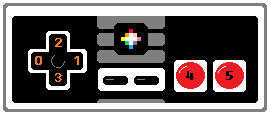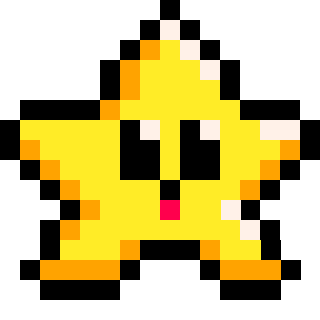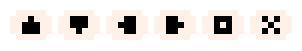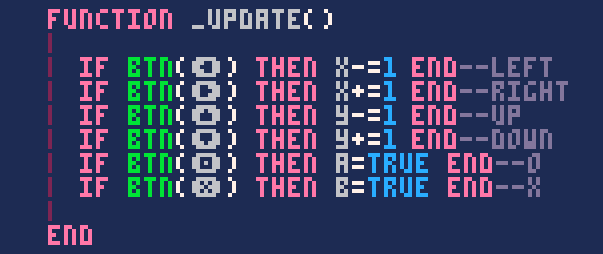# BTN

`btn` = "button"

``btn( index, [player] )``
 index a button number or button symbol (see chart below) player (optional) a player number (0-7)

If you hold the button down, this will act like it is rapidly pressed.For multiplayer games, you will need to include player numbers (0-7).

These are the button indexes you can use:

 Button Number Glyph Left 0 shift+L Right 1 shift+R Up 2 shift+U Down 3 shift+D O 4 shift+O X 5 shift+XFor Example:

``````if btn(0) then x-=1 end  --left
if btn(1) then x+=1 end  --right
if btn(2) then y-=1 end  --up
if btn(3) then y+=1 end  --down``````

It is common to see this simple style of button detection and player movement in PICO-8 games. We check if the default direction buttons are pressed using the button numbers (0-3), then if any of those are true, then we subtract or add to the X and Y position appropriately.

(Remember: The Y-axis is the opposite from what you might assume. Subtract to move upwards, and add to move downwards.)### Button GlyphsThese are the glyphs or symbols that are used inside of the PICO-8 code editor. These button glyphs can actually replace the button numbers when using the `btn()` function.

This is what that code will look like inside of PICO-8, with the buttons appearing as glyphs:Here is what the glyphs look like oustide of PICO-8:

``````function _update()

if btn(⬅️) then x-=1 end --left
if btn(➡️) then x+=1 end --right
if btn(⬆️) then y-=1 end --up
if btn(⬇️) then y+=1 end --down
if btn(🅾) then a=true end--o
if btn(❎) then b=true end--x

end``````

You can copy the code you find on this site, even if it uses these button glyphs, and paste it into PICO-8.### Multiplayer Games

In order to detect the button input for a multiplayer game, you will need to include the second argument for the player number.

``btn( button_number, player_number )``

If you understand how to use tables and loops, you can use these to help you write less code, even when you need to check for buttons of multiple players. For example, you can use a for loop to check the same button inputs for each player in one block of code:

``````num_players=2

for i=0, num_players-1 do
if btn(0,i) then
--left button pressed
--i = which player
end
end``````

One thing to keep in mind is that the player-index used in this function's second argument starts at 0, not 1. So player 1 is index 0 and player 8 is index 7. This is why the above loop starts at zero, and ends at one less than the total number of players.

 Player Count Index Number 1 2 3 4 5 6 7 8 0 1 2 3 4 5 6 7945

17 Oct 2023

`Font`